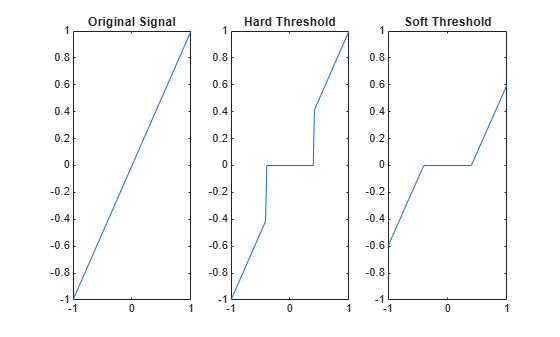# wthresh

Soft or hard thresholding

## Syntax

``Y = wthresh(X,sorh,T)``

## Description

example

````Y = wthresh(X,sorh,T)` returns the soft or hard thresholding, indicated by `sorh`, of the vector or matrix `X`. `T` is the threshold value.```

## Examples

collapse all

Generate a signal and set a threshold.

```y = linspace(-1,1,100); thr = 0.4;```

Perform hard and soft thresholding.

```ythard = wthresh(y,'h',thr); ytsoft = wthresh(y,'s',thr);```

Plot the results and compare with the original signal.

```subplot(1,3,1) plot(y,y) ylim([-1 1]) title('Original Signal') subplot(1,3,2) plot(y,ythard) ylim([-1 1]) title('Hard Threshold') subplot(1,3,3) plot(y,ytsoft) ylim([-1 1]) title('Soft Threshold')```## Input Arguments

collapse all

Input data to threshold, specified as a real-valued vector or matrix.

Data Types: `double`

Type of thresholding to perform:

• `'s'` — Soft thresholding

• `'h'` — Hard thresholding

Threshold value, specified as a positive real number.

## Output Arguments

collapse all

Thresholded data, returned as a real-valued vector or matrix. `Y` has the same dimensions as `X`.

## Algorithms

If `sorh` is `'s'`, `Y` is the soft thresholding of `X`: $\text{Y}=\text{sign(X)}·{\left(|\text{X}|-\text{T}\right)}_{+}$ where

`${\left(x\right)}_{+}=\left\{\begin{array}{ccc}x& \text{if}& x\ge 0\\ 0& \text{otherwise}& \end{array}$`

Soft thresholding is wavelet shrinkage.

If `sorh` is `'h'`, `Y` is the hard thresholding of `X`: $\text{Y}=\text{X}·{1}_{\left(|\text{X}|\text{ }\text{ }>\text{ }\text{ }\text{T}\text{ }\text{ }\right)}$ where

`${1}_{\left(|\text{X}|\text{ }\text{ }>\text{ }\text{ }\text{T}\text{ }\text{ }\right)}=\left\{\begin{array}{ccc}1& \text{if}& |\text{X}|>\text{T}\\ 0& \text{otherwise}& \end{array}$`

Hard thresholding is cruder than soft thresholding.

## Extended Capabilities

### Apps

Introduced before R2006a

## Support평가판 신청